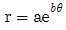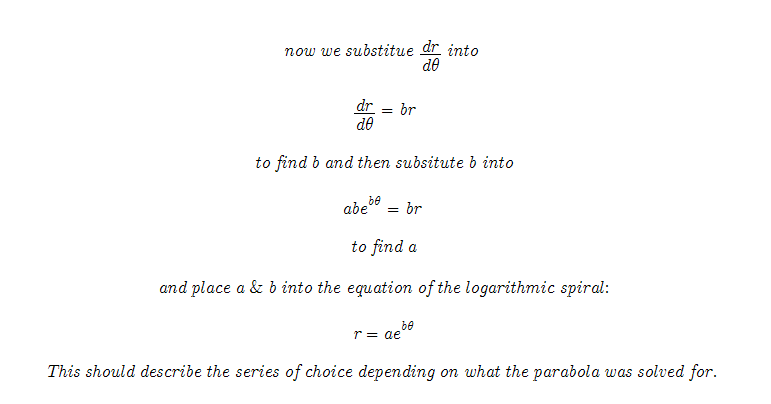Click Here to Review Past Work on Prime Numbers _______ 20070823-revised_20070724 We already have found the parabola which describes Prime numbers as they are mapped across a logarithmic spiral every Pi. We find by looking at the parabola that its equation equals the arc length of the logarithmic spiral. That is the y coordinates of the graph of the parabola. We also find that when we solve for x by the Quadratic equation that x equals the radius.Since our goal is to write an equation that describes the Prime numbers mapped on the logarithmic spiral, it is necessary to find a and b in the equation:However with a and b there are 2 unknowns. This proves to be a difficult problem to solve. However there are some tricks in our tool bag. When out of ideas: experiment. I tried to put this equation into usable form. I searched Wikipedia.org and Wolfram's "MathWorld" and found an equation that on a hunch I thought was useful.There is a bit of improvisation here. There is no direct way to relate the radius and the angle theta together in the derivative part of the equation. However with a little math tinkering, we can find dr/ds and d-theta/ds. Since they both relate to ds, we can compare there derivatives together. That is the derivative of r opposed to theta.It is important to note that this is just a "hunch." It is a summary of my best (however, incomplete) work on trying to put the Prime numbers ordered across every Pi of a logarithmic spiral into an equation. If this math holds true it could mean a great advancement in cryptography. Ideally if some of the values of the encryption algorithm were known, you could decode it with equations like these by finding the series it was encrypted with. That is wishful thinking, but it just may work. That is, a step in the right direction.   References: 1. Logarithmic Spiral from Wolfram MathWorld [equation noted with 1] 2. Wikipedia.org for equation references I will be adding more content to support this work soon. However do not wait and test the theory for yourself. May the Creative Force be with You!